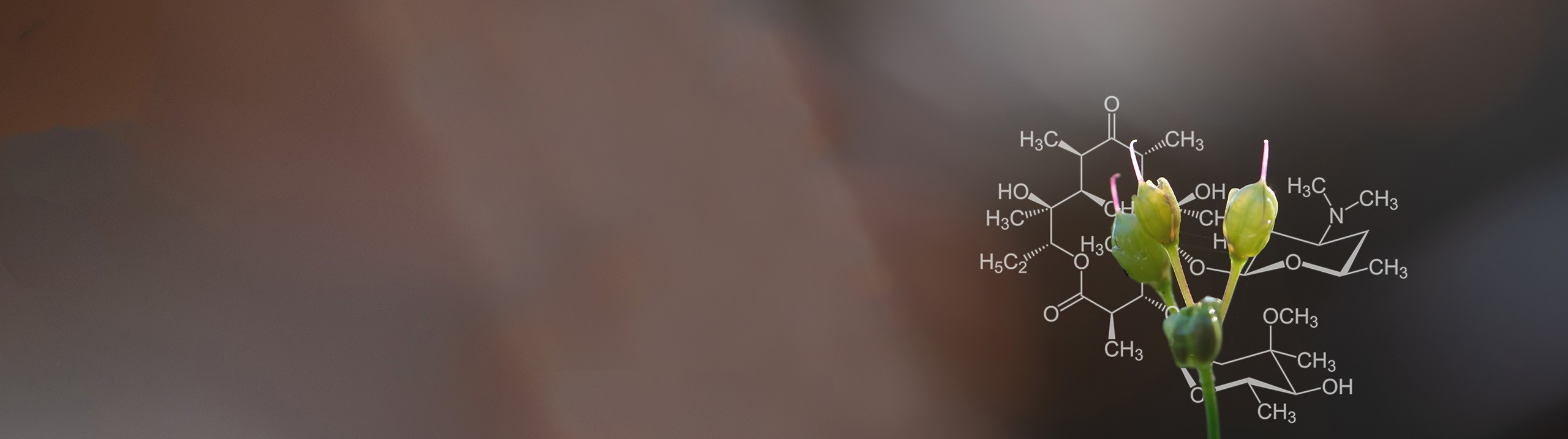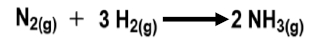Start typing, then use the up and down arrows to select an option from the list.# Biochemistry

Learn the toughest concepts covered in Biochemistry with step-by-step video tutorials and practice problems by world-class tutors

1.Introduction to Biochemistry
1

#### concept

Entropy, Thermodynamics & System vs. Surroundings3m
Play a video:
in this video, we're gonna begin our discussion on entropy. So you guys have learned in your previous courses that entropy is a measure of randomness, and we'll talk more about entropy in our next video. But before we get there, we need to first brush up on some concepts that are gonna help us better understand entropy. And the first is that entropy is a property of thermodynamics, and the laws of thermodynamics described the flows and changes of heat, energy and matter and reactions and in living things. And so, in our example below, we'll see that we have the sun here, which is the source of energy for the vast majority of living organisms on Earth. And the sun provides the solar energy that could be absorbed by plants and photosynthetic organisms. And then the photosynthetic organisms convert the solar energy into chemical energy. And then animals can eat plants to obtain the chemical energy and then to convert the chemical energy into mechanical energy or kinetic energy for their movements. And so also noticed that with each transfer of energy here, there is also a loss of energy in the form of heat. And so we'll talk more about this when we talk about the second laws of thermodynamics and are later videos. So another important thing is that thermodynamics requires a distinction between the system and the surroundings. Now the system refers to the local portion of the universe that we are focused on, whereas the surroundings refers to the rest of the universe. And so living things are systems that are referred to as biological systems, and they are a specific type of system that's known as an open system and open systems exchange both mass as well as energy with the surroundings. So let's take a look at our example and down below on the left. Here we have a living system, which is a cell, and then we have the system boundary or our biological system boundary, which is the plasma membrane, and notice that the cell is an open system, which means that it can take in Mass and also export mass with the surroundings. And it can also import energy and export energy. And so because it can exchange mass and energy with the surroundings, it is an open system. Now our system could be a biological system like an entire cell or it could be a specific chemical reaction. So here we have a reaction that occurs within most cells where glucose is converted into glucose six phosphate. So the only difference is that we have a hydroxyl group here, whereas over here we have a phosphate group. And so what you'll notice is that there is a specific enzyme that's involved here. Heck, so kindness. So it ends in a sec. So it is an enzyme. And then there are also other, uh, ions such as magnesium here, which is an essential ion of life. And then we have ATP or energy that's required for this reaction. And so you can have a specific system, uh, be a chemical reaction. And we'll see a lot of this as we move forward in our biochemistry course. And so, in our next video, we're gonna use these concepts to help us better understand entropy. So I'll see you guys in that video
2

#### concept

Understanding Entropy4m
Play a video:
So we've already said that entropy is a measure of randomness or disorder, and the greater the disorder is, the greater the entropy will be. So they go hand in hand and let's take a look at an example, and what you'll see is that we have these two billiard tables and one that's on the left notice that the billiard balls are very nicely organized. They're highly ordered, and so that creates a state of low entropy, whereas on the right billiard table, the billiard balls air scattered everywhere and they're no longer a single unit. You can see they're all over the table, and they're very disorganized and have their super highly disordered. So this creates a state of high entropy, and the reactions of the universe move the universe towards a state of maximum entropy. So the universe is moving in this direction. And so this tends to confuse students because students know that cells are able to take a bunch of amino acids and link those amino acids together to create a single protein that's highly ordered and lowers the entropy, so cells are able to essentially lower entropy. So how is it that the universe is increasing entropy. And so here's the thing recall that sells our biological systems. And in our previous video, we said that systems are a local portion of the universe that we are specifically focused on, and the deal is is that the local entropy is able to decrease as long as it's accompanied by an increase in universal entropy. And so, yes, cells are able toe decrease the local entropy. They're able to lower entropy, but they have to be accompanied. Thes reactions have to be accompanied by another reaction that ultimately ends up increasing the overall universal entropy. And we'll talk more about this in our later videos. But in this video, I really want you guys to know that high universal entropy is associated with more stability as well as lowered energy of a system. So let's take a look at this example below and of this graph and notice that the graph has free energy of the system on the why axis, as well as the reaction coordinate on the X axis or the time that passes as the reaction progresses. And so, for this particular reaction, we have reactant that have higher free energy than the products and so recall that this reaction looks somewhat like this. And so what I really want you guys to know is that the free energy of the reactions for this particular reaction is high. So here we have high energy and for the products down low, we have low energy. And so what I want you guys to know is that high energy of a system is associated with being unstable. It's unstable. It's also Mawr ordered associate it with being mawr ordered, and that is associate ID with having lower entropy. So low entropy and what you'll also notice is that low energy here is associated with being more stable. It's associated with having mawr disorder mawr disorder, and that's associated with high universal entropy. And so that's what we said earlier, that high universal entropy, which is over here on the right, is associated with more stability. So being more stable as well as having lower energy, and you can see that that's what we're seeing here. The lower energy here corresponds with all of these things, and so we'll discuss and distinguish a lot more about this when we talk about the second Law of thermodynamics and some of our later videos. But in our next video, we're going to talk about how entropy is incorporated into the Gibbs Free Energy equation. So I'll see you guys in that video.
3

#### concept

Entropy & Gibbs Free Energy Equation2m
Play a video:
So here's an equation I know you guys have definitely seen in your previous chemistry courses. And that's the Gibbs Free Energy equation, which expresses the link between changes in entropy, entropy and free energy. And so changes here is shown by a Greek symbol that's known as Delta, shown as a triangle and recall, that just means the final values minus the initial values. And so the Gibbs Free Energy equation is shown here and on the far right. We have Delta s and recall Delta s is the change in entropy of the system. So in this block will just put entropy and recall that entropy is a measure of disorder or randomness. Now T here represents temperature, but specifically, this is temperature and units of Calvin. And this tends to trick up some students because practice problems will give you guys the temperature and Celsius, and you have to be able to convert it into Calvin. So here, let's put that Calvin is equal to the degrees that are given to you and Celsius plus 273.15 So this is a nick way Asian that you guys should be able to know, to make sure your units of temperature are always in Calvin. Now, Delta H here represents the anthill p of the system. It represents the entropy. And so entropy here is, uh, the total energy of the system. So this includes the energy within every chemical bond within every molecule or compound That's part of the system. And so in this block, we can put en therapy. Now, in our last I guess variable over here is, uh, the Gibbs Free Energy represented by Delta G. So this is the change in free energy and free energy is simply the, uh, portion available to do work. So here we can put free energy. So this is on Lee, a small subset of the total energy that is actually available to perform work and by work, in a reaction. Really? All we mean is changing the components, uh, changing the concentrations of components within the system. And so again, we'll talk a lot more about this equation in our later videos. But for now, this is a good summary, and I'll see you guys in the practice problem videos
4
Problem

When solid ice water melts to liquid water, how does that affect its entropy?

5
Problem

How does the entropy change with the following reaction?6
Problem

Consider a reaction at 100˚C with ΔG = 15 KJ and ΔH = 40 KJ. Calculate the system’s change in entropy.